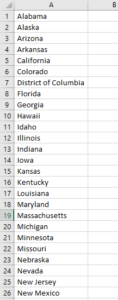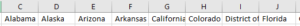# VBA Output (Print) Array to Range

Last updated on September 21, 2021

This tutorial will demonstrate how to output an array to a range using VBA.

## Output (Print) Array to Range

Data that is stored in an array can easily be outputted into an Excel sheet.  There are 3 ways to do this.

### Output Data to a Different Range

We could populate an array with data from one range in Excel, and then output the data to a different range in Excel.

``````Public Sub TestOutput()
'declare the array
Dim rnArray() As Variant
'populate the array with the range
rnArray = Range("A1:H24")
'output the array to a different range of cells
Range("J1:Q24") = rnArray()
End Sub``````

The entire array is copied in one line of code to the array, and then outputted in it’s entirety to a different range of cells on the sheet.

### Looping Through an Array and Outputting the data

This example will loop through an array, outputting the array to a range.

``````Public Sub TestLoopArray()
'declare the array
Dim rnArray() As Variant
'Declare the integer to store the number of rows
Dim iRw As Integer
'Assign range to a the array variable
rnArray = Range("A1:A10")
'loop through the values in the array
For iRw = LBound(rnArray) To UBound(rnArray)
'populate a different range with the data
Cells(iRw, 2).Value = rnArray(iRw, 1)
Next iRw
End Sub``````

LBound and UBound returns the Starting index (Lower Bound) and Ending index (Upper Bound) of an array, in this case 1 and 10.

### Transpose Array Data

We can also transpose the data in the Array to the Excel sheet.  Transpose allows you to display the data horizontally across the Excel sheet.

For example, if we have a list of states in Excel and wish to transpose them.We could then run the following code:

``````Public Sub TestOutputTranspose()
'declare the array
Dim rnArray() As Variant
'populate it with the range
rnArray = Range("A1:A38")
'transpose the data
Range(Cells(1, 3), Cells(1, 40)).Value = Application.Transpose(rnArray)
End Sub``````

Which would result in the following:## Output to Debug.Print

We can also output the array values to the debug window.

``````Public Sub TestLoopArray()
'declare the array
Dim rnArray() As Variant
'Declare the integer to store the number of rows
Dim iRw As Integer
'Assign range to a the array variable
rnArray = Range("A1:A10")
'loop through the rows - 1 to 10
For iRw = 1 To UBound(rnArray)
'output to the immediate window
Debug.Print rnArray(iRw, 1)
Next iRw
End Sub``````

Stop searching for VBA code online. Learn more about AutoMacro - A VBA Code Builder that allows beginners to code procedures from scratch with minimal coding knowledge and with many time-saving features for all users!